# Trio ratio

Hans, Alena, and Thomas have a total of 740 USD. Hans and Alena split in the ratio 5: 6 and Alena and Thomas in the ratio 4: 5. How much will everyone get?

a =  240
h =  200
t =  300

### Step-by-step explanation:

h+a+t = 740
6h = 5a
5a = 4t

h+a+t = 740
6•h = 5•a
5•a = 4•t

a+h+t = 740
5a-6h = 0
5a-4t = 0

a = 240
h = 200
t = 300

Our linear equations calculator calculates it.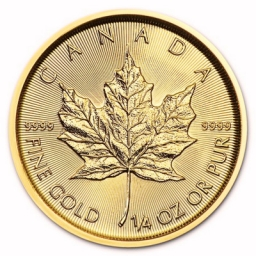Did you find an error or inaccuracy? Feel free to write us. Thank you!Tips to related online calculators
Need help to calculate sum, simplify or multiply fractions? Try our fraction calculator.
Check out our ratio calculator.
Do you have a linear equation or system of equations and looking for its solution? Or do you have a quadratic equation?

## Related math problems and questions:

• Revenue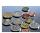Mr. Robert and Mr. Norbert get together € 1400. The revenue split in the ratio of 4:3, depending upon each work. How many got each of them?
• Honza + Alice + Tonda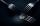Honza + Alice + Tonda have a total of 111 USD. The ratio between Honza and Alena is 5:6 and the ratio between Alice and Tonda is 4:5 How many money have each of them?
• CoinsThe money - coins are minted from the hardest bronze, which contains copper and tin in a ratio of 41: 9. How much copper and tin are in 2kg of bronze money?
• Ratio6 numbers are in the ratio 1:5:1:5:5:5. Their sum is 242. What are the numbers?
• Composite ratioJakub, Aneta, and Lenka divided 1342 USD in the ratio 5/2: 3/10: 1/4. How much did Lenka take?
• BonusThe gross wage was 1323 USD including 25% bonus. How many USD were bonuses?
• Division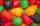Three siblings Helena, Oliver and George split the bag with candies on merit in the ratio 6:1:4. How many candies should each get if in bag were 88?
• Moneys in triple ratioMilan, John and Lili have a total 344 euros. Their amounts are in the ratio 1:2:5. Determine how much each of them has?
• BanknotesEva deposit 7800 USD in 50 banknotes in the bank. They had a value of 100 USD and 200 USD. How many were they?
• Unknown amount of money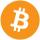Damian and Denis split an unknown amount in the ratio of 5:4. Damian got six euros more than Denis. Calculate an unknown amount. Determine how much money got Damian and how Denis.
• Sharing moneyA certain sum of money is shared in the ratio 2 :3:9. If the difference between the first and the second is \$40, then the amount of money shared is
• 925 USDFour classmates saved an annual total 925 USD. The second save twice as the first, third 35 USD more than the second and fourth 10 USD less than the first. How USD save each of them?
• Tyreek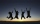Tyreek, Antuan and Alex are given a total of \$3500 to be shared among themselves in the ratio 3:5:12. How much each get money?
• Three 43Three brothers inherited a cash amount of 62,000, and they divided it among themselves in the ratio of 5:4:1. How much more is the largest share than the smallest share?
• EurosPeter, Jane, and Thomas have together € 550. Tomas has 20 euros more than Jane, Peter € 150 less than Thomas. Determine how much has each of them.
• Bottle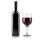Bottle with wine costs 42 USD. Wine is 11 USD more expensive than the empty bottle. How much is the bottle itself?
• TwelveTwelve students work on a week forestry brigade. One hundred spruces will receive x CZK, one hundred pine y CZK. How many receive each one students did in one day if they planted a total of 25,000 spruces per week and 30,000 pine trees? Express by express